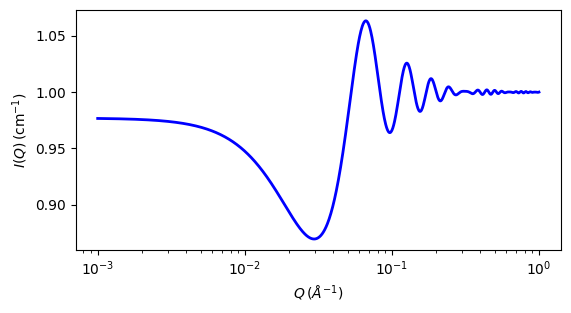# squarewell

Square well structure factor with Mean Spherical Approximation closure

Parameter

Description

Units

Default value

effective radius of hard sphere

50

volfraction

volume fraction of spheres

None

0.04

welldepth

depth of well, epsilon

kT

1.5

wellwidth

width of well in diameters (=2R) units, must be > 1

diameters

1.2

The returned value is a dimensionless structure factor, $$S(q)$$.

Calculates the interparticle structure factor for a hard sphere fluid with a narrow, attractive, square well potential. The Mean Spherical Approximation (MSA) closure relationship is used, but it is not the most appropriate closure for an attractive interparticle potential. However, the solution has been compared to Monte Carlo simulations for a square well fluid and these show the MSA calculation to be limited to well depths $$\epsilon < 1.5$$ kT and volume fractions $$\phi < 0.08$$.

Positive well depths correspond to an attractive potential well. Negative well depths correspond to a potential “shoulder”, which may or may not be physically reasonable. The stickyhardsphere model may be a better choice in some circumstances.

Computed values may behave badly at extremely small $$qR$$.

Note

Earlier versions of SasView did not incorporate the so-called $$\beta(q)$$ (“beta”) correction  for polydispersity and non-sphericity. This is only available in SasView versions 5.0 and higher.

The well width $$(\lambda)$$ is defined as multiples of the particle diameter $$(2 R)$$.

The interaction potential is:

$\begin{split}U(r) = \begin{cases} \infty & r < 2R \\ -\epsilon & 2R \leq r < 2R\lambda \\ 0 & r \geq 2R\lambda \end{cases}\end{split}$

where $$r$$ is the distance from the center of a sphere of a radius $$R$$.

In SasView the effective radius may be calculated from the parameters used in the form factor $$P(q)$$ that this $$S(q)$$ is combined with.

For 2D data: The 2D scattering intensity is calculated in the same way as 1D, where the $$q$$ vector is defined as

$q = \sqrt{q_x^2 + q_y^2}$Fig. 128 1D plot corresponding to the default parameters of the model.

Source

squarewell.py

References

1. R V Sharma, K C Sharma, Physica, 89A (1977) 213

2. M Kotlarchyk and S-H Chen, J. Chem. Phys., 79 (1983) 2461-2469

Authorship and Verification

• Author: